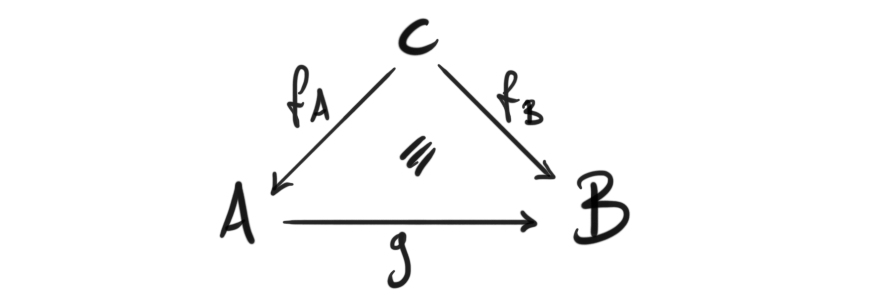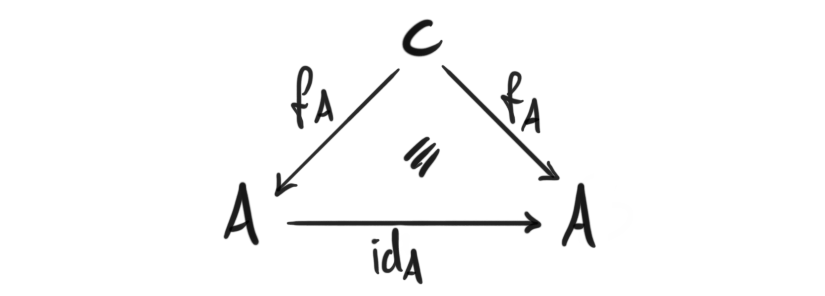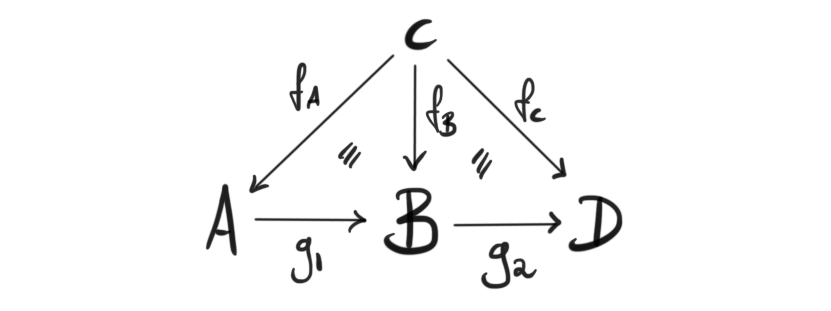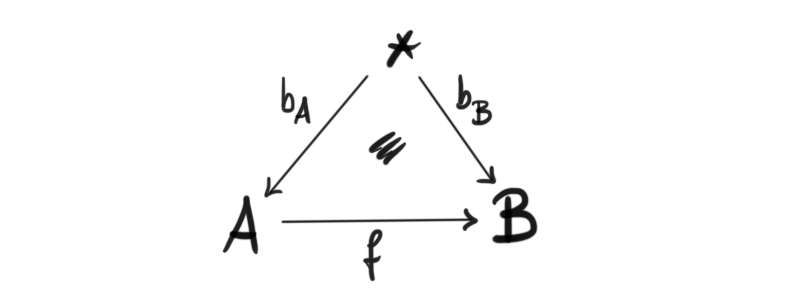Since one of my main mathematical interests is homotopy theory, im bound to often bump into things that require the use of base-points. This has long been the classical way to study spaces, especially in terms of homotopy groups. When I was introduced to these so-called pointed spaces, I couldn’t help but feel that these we less natural, or more ad hoc, than regular spaces. I didn’t know much about categories then, but have since learned it is usually in this context that some form of naturality occur. It turns out that pointed spaces actually come from a very nice natural categorical construction, which of course is the focus of this post. I will assume introductory knowledge of categories, and I will try to keep this short for once.

## Definition#

As the name implies, we will have something like “a category under some object”, but we will have to make this precise of course. Under categories are also sometimes called coslice categories, and they are in fact a special case of a construction called comma categories, which we will not cover today. The idea is roughly to fix an object, and study morphisms out of said fixed object instead the object itself. This will hopefully be clear from the definition.

Definition (Under category): Let $\mathcal{C}$ be a category, and $c$ an object in it. The under category $c\downarrow \mathcal{C}$ is a category whose objects are all morphisms $f_A: c\rightarrow A$ that start at $c$, and whose morphisms are commuting triangles of the formIt then (at least for me) makes intuitively sense that we call it the under category, since it consists off all things happening “under” an object $c$ in some nice way. It is in fact a category, since it has identity morphismsfor any object $f:c\rightarrow A$ in the category, and composition of morphisms are associative because the commutivity of the inner triangles in the following diagram imply the commutivity of the outer triangle.This is all we need in order to call $c\downarrow \mathcal{C}$ a category.

## Examples#

Our motivation was to create pointed topological spaces, so how do we get these as an under category? The category of pointed topological spaces with base-point preserving maps, is precisely the category $\ast \downarrow Top$ of topological spaces under a one point space! How can we see this? Any object in the under category is a map $b_A$ from a point to a space $A$. Call the image $x=b_A(\ast)$ of this map the base-point of the space $X$. This information is exactly the same as having a space with a specified point $(X, x)$, i.e. an object in the category of pointed topological spaces. Also, maps in this under category are maps that commute with the formation of these base-points, i.e. diagramswhich in terms of just the pointed spaces are maps that preserve the chosen base-points in the two spaces $A$ and $B$, because $f(b_A(\ast))=b_B(\ast)$, i.e. base-point preserving maps. Hence we have a nice categorical description of pointed spaces, and they are an example of a very natural construction. Neat!

Another example is the category of algebras over a ring. To recap the definition, an algebra over a ring $R$, also called an $R$-algebra, is a ring $A$ together with a ring map $s_A: R\rightarrow A$, making it into an $R$-module in a compatible way. This is maybe starting to look familiar to the previous example. An $R$-algebra morphism is, now not surprisingly, a ring map between two $R$-algebras, such that it commutes with their respective map from $R$, i.e. a map such thatcommutes. Hence, the category of $R$-algebras, is the under category $R\downarrow Rings$ in the category of rings.

It said I would keep this short, so I wont say anything more for now. I will mention that the semester has started again, so not much time to write on the blog… But, I have started writing my master thesis, so maybe Ill do some writing here about what I write about there, or some progress updates etc. Time will tell.